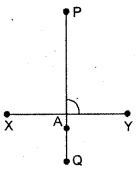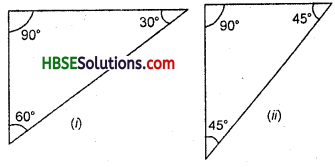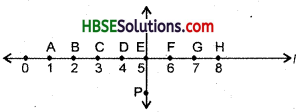# HBSE 6th Class Maths Solutions Chapter 5 Understanding Elementary Shapes Ex 5.5

Haryana State Board HBSE 6th Class Maths Solutions Chapter 5 Understanding Elementary Shapes Ex 5.5 Textbook Exercise Questions and Answers.

## Haryana Board 6th Class Maths Solutions Chapter 5 Understanding Elementary Shapes Exercise 5.5

Question 1.
Which of the following are models for perpendicular lines :
(a) The adjacent edges of a table top.
(b) The lines of a railway track.
(c) The line segments forming the letter U.
(d) The letter C.
Solution:
(a) The adjacent edges of a table and
(c) The line segments forming the letter ‘L’.Question 2.
Let $$\overline{\mathrm{PQ}}$$ be the perpendicular to the line segment $$\overline{\mathrm{XY}}$$. Let $$\overline{\mathrm{PQ}}$$ and $$\overline{\mathrm{XY}}$$ intersect in the point A.
(a) How is the point A placed in $$\overline{\mathrm{XY}}$$ ?
(b) What is the mea¬sure of ∠PAY ?Solution:
(a) Point A lies between X and Y.
(b) Measure of ∠PAY = 90°.

Question 3.
There are two “set squares” in your box.
What are the measures of the angles that are formed at their corners ? Do they have any angle measure that is common?
Solution:(i) 90°, 60° and 30°,
(ii) 90°, 45° and 45°, common angle = 90°.Question 4.
Study the diagram. The line T is perpendicular to line im\
(a) Is CE = EG ?
(b) Does PE bisect CG ?
(c) Identify any two line segments for which PE is the perpendicular bisector.
(d) Are these true ?
(i) AC > FG, (ii) CD = GH, (Hi) BC < EH.Solution:
(a) Yes, CE = EG. .
(b) Yes, PE bisects CG.
(c) PE is the perpendicular bisector of DF and BH.
(d) (i) T, (ii) T, (Hi) T.